### Home > CALC > Chapter 4 > Lesson 4.1.1 > Problem4-6

4-6.
1.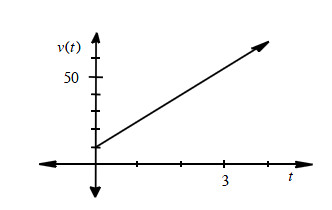While driving to work, Mr. Matlack's velocity was v(t) = 15t + 10, where t is hours and v(t) is miles per hour. Determine how far Mr. Matlack lives from school if it takes him: Homework Help ✎

1. 1 hour to get to work.

2. 4 hours to get to work.

3.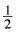hour to get to work.

4. t hours to get to work.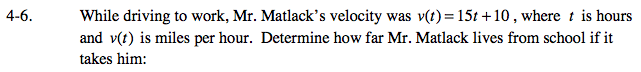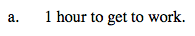Area under the curve is a trapezoid.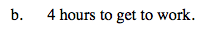Refer to hint in part (a).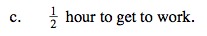Refer to hint in part (a).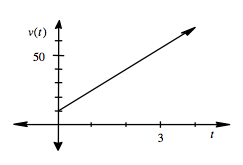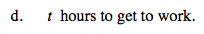Unlike parts (a) - (c), you are now being asked to find a distance function, rather than a finite distance.

$s(t)=\frac{1}{2}h(b_{1}+b_{2})=\frac{1}{2}t(v(0)+v(t))=$